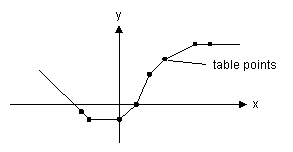﻿ 20-sim webhelp > Language Reference > Functions > External > table

# table

## Syntax

y = table(file_name,x);

## Description

This model uses a one-dimensional table with data points to calculate the output y = f(x) as a function of the input x. The output y is calculated using linear interpolation between the table data points.

 • The input data x of the first column needs to be monotonically increasing.
 • Discontinuities are allowed, by providing the same input point twice in the table.
 • Values outside of the table range, are computed by linear extrapolation of the last two points.

The first argument (file_name) of this function must be a parameter of the type string and is used as a reference to a filename. This reference can be specified using the complete path or relative to the model directory. The second argument (x) should be a real variable.

 table   -2.5 -2 0 1 2 3 5 6 (example):   -0.5 -1 -1 0 2 3.0 4 4.0A table must be stored as an ASCII (text) file or a Comma Separated Values (.csv) file and should consist two columns of data. The first column consists of the x-values and the second column of the corresponding y-values. Each line of the file may only contain one x- and one corresponding y-value. The two values must be separated by a space or a tab. Each new set of x- and y-values must start on a new line. No comment or other text may be part of the table-file.

## Example

parameters

string filename = 'data.txt';

variables

real y;

real x;

equations

x = ramp(1);

y = table(filename,x);

Example data.txt format:

-2.5, -0.5

-2.0, -1.0

0.0, -1.0

1.0, 0.0

2.0, 2.0

3.0, 3.0

5.0, 4.0

6.0, 4.0

## Limitations

x and y must be scalars. from_file must be string parameter.